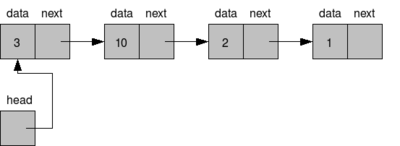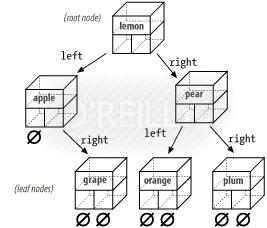Associative Arrays

Associative arrays use scalar values as subscripts, which means you can have a word represent the index value. Perl uses the percent sign (%) to distinguish an associative array from an ordinary array variable.

 Referring to Associative array elements \$fruit{"bananas"} = 1; \$number{3.14159}; \$integer{-7}; \$fruit{\$my_fruit}; Note: using the dollar sign (\$) tells the perl interpreter that this is a single scalar item and is to be treated as such Creating an associative array %associative_array;                          # declare an associative array %array = ("foo", "26", "bar", "27");         # declare and populate an associative array \$fruit{"bananas"} = 1; \$fruit{"apples"} = 3; foreach \$item (sort keys (%fruit)) {    print \$item . " = " . \$fruit{\$item} . "\n"; } Note: There is no order within the array, elements can be all over the place Copying associative arrays from array variables @fruit = (apples", 6, "cherries", 8, "oranges", 11); %fruit = @fruit; Adding an element \$fruit{"bananas"} = 1; Deleting an element delete(\$fruit{"bananas"}); Note: delete is the only way to delete from an associative array Listing the keys %array = ("foo", "26", "bar", "27"); @keys = keys(%array); print("@keys\n"); # keys is commonly used as below foreach \$i (keys (%array)) {    print \$i . "\n"; } Note: in no particular order will the list be returned Listing the values %array = ("foo", "26", "bar", "27"); @values = values(%array); print("@values\n"); foreach \$i (values (%array)) {    print \$i . "\n"; } Note: in no particular order will the list be returned Looping an associative array %valle = ("Paul", 35, "Lorraine", "31", "Dominic", 12, "Jessica", 8); ## Using the foreach loop foreach \$i (keys (%valle)) {    print \$i . " " . \$valle{\$i} . "\n"; } # Using a while loop while ((\$name, \$age) = each(%valle)) {    print \$name . " " . \$age . "\n";    ## delete \$valle{"Paul"};               ## Do not use delete as it makes each unpredictable } Note: do not use delete when using each, because the behavior of each unpredictable

For more on manipulating arrays see List Manipulation.

Data Structures

You can use associative arrays to simulate a wide variety of data structures found in high-level languages, you could implement the following data structures

• Structures
• Trees
• Databases

A Linked List is a simple data structure that enables you to store items in a particular order. Each element of the linked list contains two fields

• The value associated with this element
• A reference, or pointer to the next element in the list.
• A special header variable points to the first element in the listStructures

Many programming languages enable you to define collections of data called structures. Like Lists, structures are collections of values, each element of a structure, however, has its own name and can be accessed by that name.

 Simulate a structure # C Structure struct {    int field1;    int field2;    int field3; } mystructure; # Perl Simulated structure %mystructure = ( "field1", "",                  "field2", "",                  "field3", "");

Trees

A tree is similar to a linked list, except that each element of a tree points to more than one other element. The simplest example of a tree is a binary tree. Each element of a binary tree, called a node, points to two other elements, called the left child and right child. Each of these children points to two children of its own and so on, the very last node are called leaf nodes.The following terminology is used when describing trees

• Because each children of a node is a tree of its own, the left child and the right child are often called the left subtree and the right subtree of the node
• The first node of the tree is called the root of the tree
• Nodes that have no children are called leaf nodes.
 Tree Example \$rootname = "parent"; %tree = ("parentleft", "child1",          "parentright", "child2",          "child1left", "grandchild1",          "child1right", "grandchild2",          "child2left", "grandchild3",          "child2right", "grandchild4"); # traverse tree, printing its elements &print_tree(\$rootname); sub print_tree {    local (\$nodename) = @_;    local (\$leftchildname, \$rightchildname);    \$leftchildname = \$nodename . "left";    \$rightchildname = \$nodename . "right";    if (\$tree{\$leftchildname} ne "") {       &print_tree(\$tree{\$leftchildname});    }    print ("\$nodename\n");    if (\$tree{\$rightchildname} ne "") {       &print_tree(\$tree{\$rightchildname});    } }

Databases

Using the above methods you can build quite complex databases, associative arrays can use variable records lengths, are accessible either sequentially or randomly.

The below calculator example is a very complex script, it uses associative arrays and recursive subroutines and the tree structure.

Calculator Example:

 To run the calculator program \$calculator 11 + 5 * (4 - 3) ^D Should result in 16 very complex example ```# statements which initialize the program \$nextnodenum = 1; # initialize node name generator &get_next_item; # read first value from file \$treeroot = &build_expr; \$result = &get_result (\$treeroot); print ("the result is \$result\n"); # Build an expression. sub build_expr { local (\$currnode, \$leftchild, \$rightchild); local (\$operator); \$leftchild = &build_add_operand; if (&is_next_item("+") || &is_next_item("-")) { \$operator = &get_next_item; \$rightchild = &build_expr; \$currnode = &get_new_node (\$operator, \$leftchild, \$rightchild); } else { \$currnode = \$leftchild; } } # Build an operand for a + or - operator. sub build_add_operand { local (\$currnode, \$leftchild, \$rightchild); local (\$operator); \$leftchild = &build_mult_operand; if (&is_next_item("*") || &is_next_item("/")) { \$operator = &get_next_item; \$rightchild = &build_add_operand; \$currnode = &get_new_node (\$operator, \$leftchild, \$rightchild); } else { \$currnode = \$leftchild; } } # Build an operand for the * or / operator. sub build_mult_operand { local (\$currnode); if (&is_next_item("(")) { # handle parentheses &get_next_item; # get rid of "(" \$currnode = &build_expr; if (! &is_next_item(")")) { die ("Invalid expression"); } &get_next_item; # get rid of ")" } else { \$currnode = &get_new_node(&get_next_item, "", ""); } \$currnode; # ensure correct return value } # Check whether the last item read matches # a particular operator. sub is_next_item { local (\$expected) = @_; \$curritem eq \$expected; } # Return the last item read; read another item. sub get_next_item { local (\$retitem); \$retitem = \$curritem; \$curritem = &read_item; \$retitem; } # This routine actually handles reading from the standard # input file. sub read_item { local (\$line); if (\$curritem eq "EOF") { # we are already at end of file; do nothing return; } while (\$wordsread == @words) { \$line = ; if (\$line eq "") { \$curritem = "EOF"; return; } # the following two lines fix a problem # in the example as printed in the book \$line =~ s/\(/ ( /g; \$line =~ s/\)/ ) /g; \$line =~ s/^\s+|\s+\$//g; @words = split(/\s+/, \$line); \$wordsread = 0; } \$curritem = \$words[\$wordsread++]; } # Create a tree node. sub get_new_node { local (\$value, \$leftchild, \$rightchild) = @_; local (\$nodenum); \$nodenum = \$nextnodenum++; \$tree{\$nodenum} = \$value; \$tree{\$nodenum . "left"} = \$leftchild; \$tree{\$nodenum . "right"} = \$rightchild; \$nodenum; # return value } # Calculate the result. sub get_result { local (\$node) = @_; local (\$nodevalue, \$result); \$nodevalue = \$tree{\$node}; if (\$nodevalue eq "") { die ("Bad tree"); } elsif (\$nodevalue eq "+") { \$result = &get_result(\$tree{\$node . "left"}) + &get_result(\$tree{\$node . "right"}); } elsif (\$nodevalue eq "-") { \$result = &get_result(\$tree{\$node . "left"}) - &get_result(\$tree{\$node . "right"}); } elsif (\$nodevalue eq "*") { \$result = &get_result(\$tree{\$node . "left"}) * &get_result(\$tree{\$node . "right"}); } elsif (\$nodevalue eq "/") { \$result = &get_result(\$tree{\$node . "left"}) / &get_result(\$tree{\$node . "right"}); } elsif (\$nodevalue =~ /^[0-9]+\$/) { \$result = \$nodevalue; } else { die ("Bad tree"); } }```